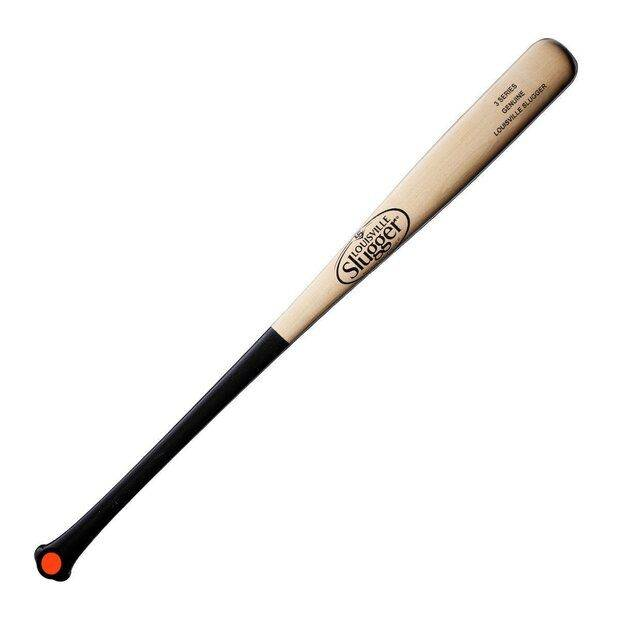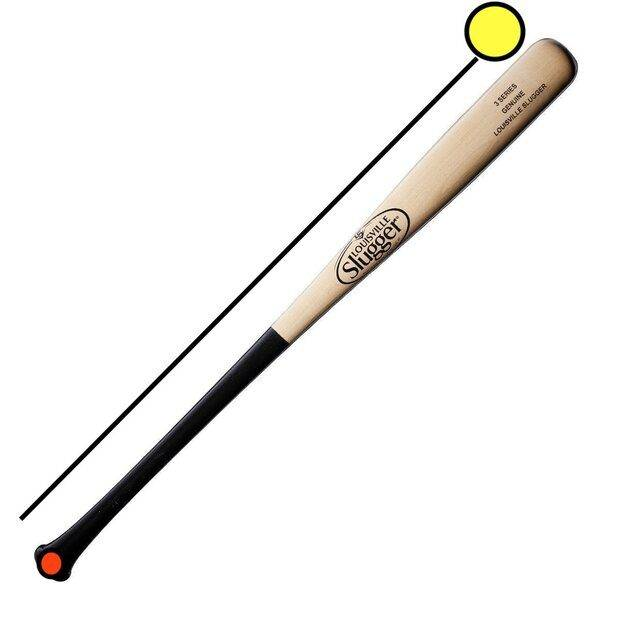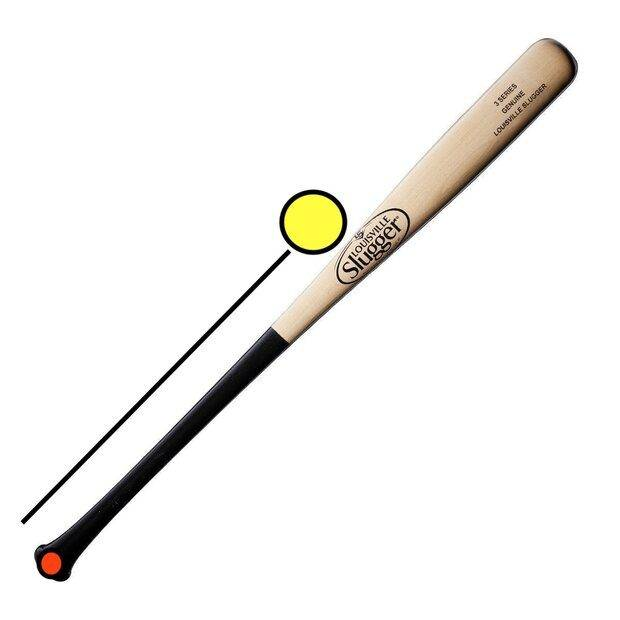# Impulse and distance from the axis of rotation

crudux_cruo
I'm trying to model the linear collision of a bat and a ball using the conservation of angular momentum. The ball is a point particle with at rest wrt the axis of rotation, and the bat is being treated as a rod of negligible radius. I have had to work through several problems involving a ball with a given linear momentum hitting a pole and finding the resulting angular momentum of the system, but I can't find any information on the reverse.

The red dot marks the axis of rotationMy intuition says that the farther away from the handle that the bat hits the ball, the more force will be imparted on the ball. I cannot find anything to support this, and when I try the math myself I get very confusing results.

For reference I assume the bat has zero momentum after the collision, and so all momentum is transferred to the ball. If the ball (the yellow circle) is here, it has a given moment of inertia.However if the ball is closer to the handle, it would have less rotational inertia. This would mean that it would have greater angular velocity. Converting it to linear velocity shows the same trend.I am almost positive that I am making a fundamental mistake on what I am sure is a painfully simple problem, but I cannot make sense of it. I am alright with challenging my intuition, but I'm not sure if I am going about it the right way.

Homework Helper
2022 Award
What exactly are you trying to calculate? What are the initial conditions? Can the ball rotate? Is the collision elastic?

have had to work through several problems involving a ball with a given linear momentum hitting a pole and finding the resulting angular momentum of the system, but I can't find any information on the reverse.
Start at the bottom line and read upwards! I don't understand this issue...an equation is an equation.

crudux_cruo
What exactly are you trying to calculate? What are the initial conditions? Can the ball rotate? Is the collision elastic?

Start at the bottom line and read upwards! I don't understand this issue...an equation is an equation.
I apologize for the low quality post. I will figure it out another way.

Homework Helper
2022 Award
•russ_watters
crudux_cruo
I formulated the problem using conservation of angular momentum and treated the system as objects with moment of inertia as stated above.

I am basing my logic off the logic that was used in a textbook. Angular collisions were never described in any detail in that book, and my ability to parse things together secondhand has not filled whatever gap there seems to be.

I am not sure how else to reformulate my question, and the fact that this is a consistent problem when posting on here tells me that my posts are low quality.

Last edited:
Homework Helper
2022 Award
I'm happy to help but that is your call...

•russ_watters and crudux_cruo
crudux_cruo
I genuinely appreciate it, and I apologize that I cannot communicate more effectively otherwise I would consider the offer. I hope that you enjoy the rest of your day.

crudux_cruo
I'm happy to help but that is your call...
I realize that my responses were flustered and needlessly rude, so I apologize. I am going to take you up on your offer if you are still willing, but first I am going to figure out how to best reexpress my question. I think I am making the mistake of talking strictly about my intuition and being very vague about the actual physics.

Again, sorry for the earlier attitude. I'd hate to respond to your genuine attempt at help by acting chastised.

crudux_cruo
I'm happy to help but that is your call...
I am having trouble relating torques and forces. I can convert a force to a torque, but I am having difficulty conceptualizing how I can do the reverse (in terms of when it would be appropriate to actually do that).

I asked about the baseball bat hypothetical because I was curious if there was an optimum place for the bat to hit the ball. Purely idealized hypothetical, I am speaking purely and entirely in terms of torques and forces.

Does the bat apply a greater force to the ball the further away the collision occurs from the axis of rotation (in this case, the handle)?

It's not a perfectly inelastic collision, the ball is stationary and the bat has a given angular momentum. The collision occurs and the bat is no longer moving and the ball must now have that same angular momentum. Doing the math and treating the ball as a point particle, the ball seems to have less moment of inertia the closer it is to the handle and thus more angular velocity. The ball is not rotating upon itself.

I have struggled thinking about how I can best phrase this question, so if I am still not clear please tell me and I will reiterate where I may be failing. I am trying to use the hypothetical in bold to arrive at an answer for my question that's italicized. Instead I am confusing myself and my intuition.

I hope I make a little more sense now, but if I am just repeating earlier points let me know and I will happily try again.

Leo Liu
I am having trouble relating torques and forces. I can convert a force to a torque, but I am having difficulty conceptualizing how I can do the reverse (in terms of when it would be appropriate to actually do that).
To my knowledge, I don't think you can find the force from the torque without specifying a direction for the force. You can use vector product to calculate torque ##\mathbf{\mathcal T}=\mathbf r\times\mathbf F##. However, its reverse operation will not give you a definitive direction for the force. Therefore it is impossible to infer both the direction or the angle the force vector makes with the arm vector and the magnitude of the force vector from the direction and the length of the torque created.